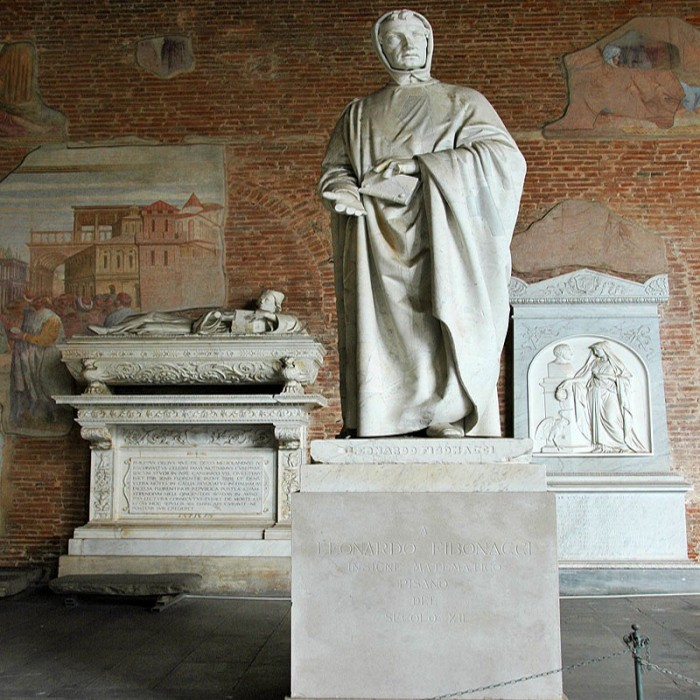# Fibonacci (c. 1175 – c. 1250)

Italian

Leonardo Bonacci (c. 1175 – c. 1250)—known as Fibonacci, and Leonardo of Pisa, Leonardo Pisano Bigollo, Leonardo Fibonacci—was an Italian mathematician, considered to be "the most talented Western mathematician of the Middle Ages".

Fibonacci popularized the Hindu–Arabic numeral system in the Western World primarily through his composition in 1202 of Liber Abaci (Book of Calculation).

He also introduced Europe to the sequence of Fibonacci numbers, which he used as an example in Liber Abaci.

Fibonacci was born around 1175 to Guglielmo Bonacci, a wealthy Italian merchant and, by some accounts, the consul for Pisa.

Fibonacci travelled with him as a young boy, and it was in Bugia (now Béjaïa, Algeria) that he learned about the Hindu–Arabic numeral system.

Fibonacci travelled extensively around the Mediterranean coast, meeting with many merchants and learning about their systems of doing arithmetic.

He soon realised the many advantages of the Hindu-Arabic system.

In 1202, he completed the Liber Abaci (Book of Abacus or Book of Calculation) which popularized Hindu–Arabic numerals in Europe.

Fibonacci became a guest of Emperor Frederick II, who enjoyed mathematics and science.

In 1240, the Republic of Pisa honored Fibonacci by granting him a salary in a decree that recognized him for the services that he had given to the city as an advisor on matters of accounting and instruction to citizens.

The date of Fibonacci's death is not known, but it has been estimated to be between 1240 and 1250, most likely in Pisa.

Liber Abaci posed, and solved, a problem involving the growth of a population of rabbits based on idealized assumptions. The solution, generation by generation, was a sequence of numbers later known as Fibonacci numbers.

In the 19th century, a statue of Fibonacci was constructed and raised in Pisa. Today it is located in the western gallery of the Camposanto, historical cemetery on the Piazza dei Miracoli.

There are many mathematical concepts named after Fibonacci because of a connection to the Fibonacci numbers. Examples include the Brahmagupta–Fibonacci identity, the Fibonacci search technique, and the Pisano period.

Beyond mathematics, namesakes of Fibonacci include the asteroid 6765 Fibonacci and the art rock band The Fibonaccis.